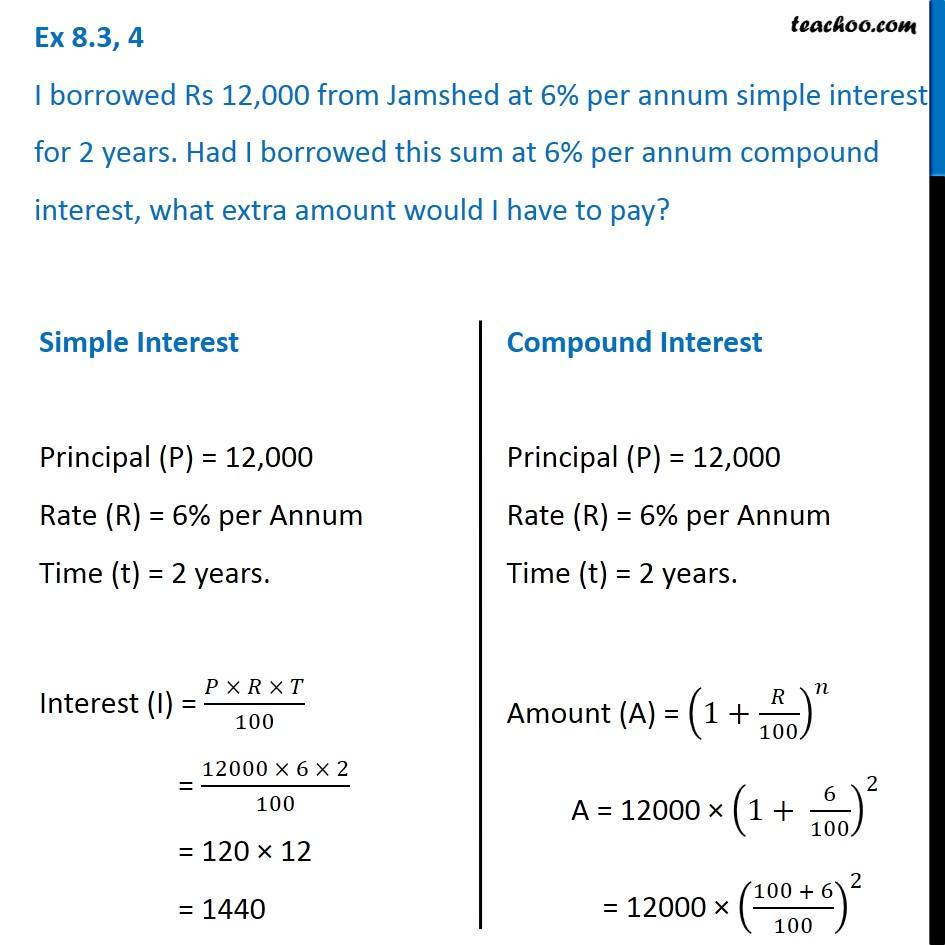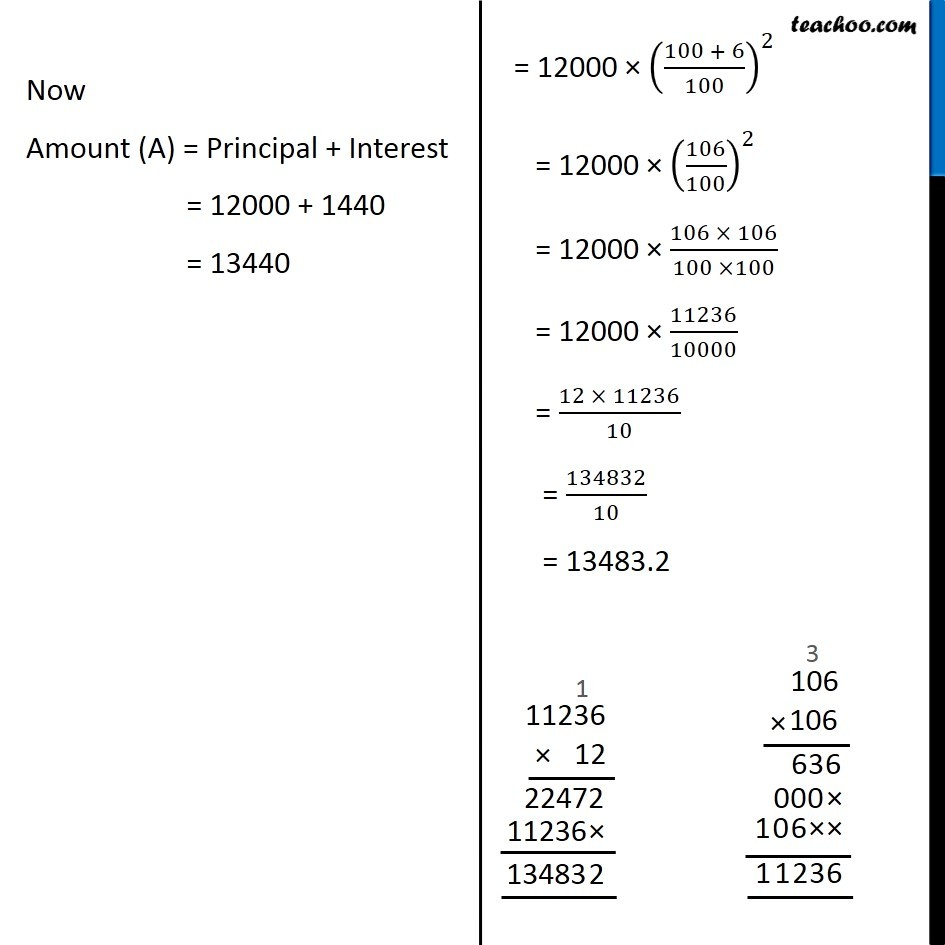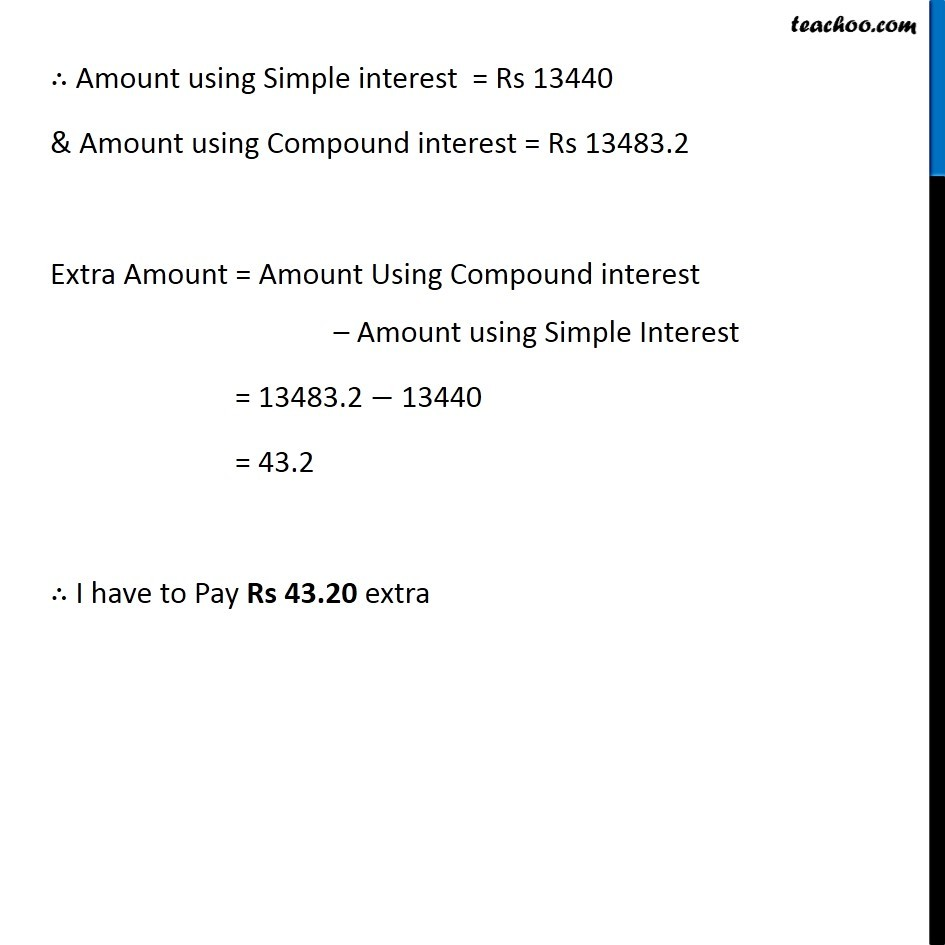Ex 7.3

Chapter 7 Class 8 Comparing Quantities
Serial order wiseLearn in your speed, with individual attention - Teachoo Maths 1-on-1 Class

### Transcript

Question 4 I borrowed Rs 12,000 from Jamshed at 6% per annum simple interest for 2 years. Had I borrowed this sum at 6% per annum compound interest, what extra amount would I have to pay?Simple Interest Principal (P) = 12,000 Rate (R) = 6% per Annum Time (t) = 2 years. Interest (I) = (𝑃 × 𝑅 × 𝑇)/100 = (12000 × 6 × 2)/100 = 120 × 12 = 1440 Compound Interest Principal (P) = 12,000 Rate (R) = 6% per Annum Time (t) = 2 years. Amount (A) = (1+𝑅/100)^𝑛 A = 12000 × (1+ 6/100)^2 = 12000 × ((100 + 6)/100)^2 Now Amount (A) = Principal + Interest = 12000 + 1440 = 13440 = 12000 × ((100 + 6)/100)^2 = 12000 × (106/100)^2 = 12000 × (106 × 106)/(100 ×100) = 12000 × 11236/10000 = (12 × 11236)/10 = 134832/10 = 13483.2 ∴ Amount using Simple interest = Rs 13440 & Amount using Compound interest = Rs 13483.2 Extra Amount = Amount Using Compound interest – Amount using Simple Interest = 13483.2 − 13440 = 43.2 ∴ I have to Pay Rs 43.20 extra